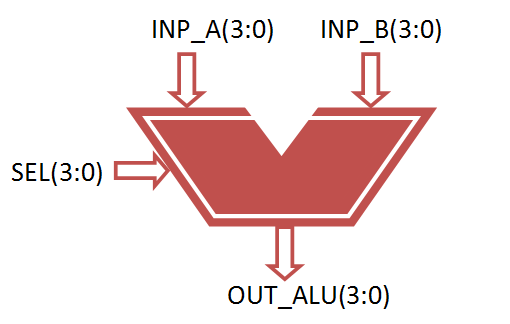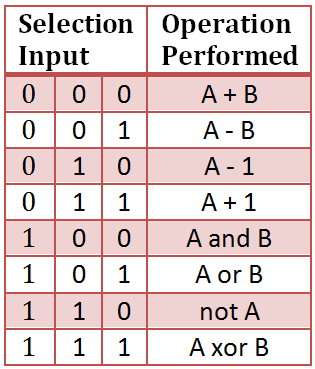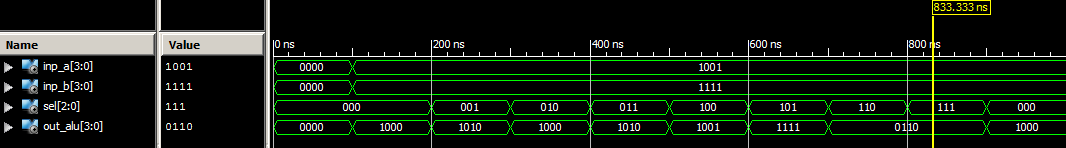# VHDL code for 4-bit ALU

### ALU

ALU’s comprise the combinational logic that implements logic operations such as AND, OR, NOT gate and arithmetic operations, such as Adder, Subtractor.

Functionally, the operation of typical ALU is represented as shown in diagram below,

###Functional Description of 4-bit Arithmetic Logic Unit

Controlled by the three function select inputs (sel 2 to 0), ALU can perform all the 8 possible logic operations### VHDL Code for 4-bit ALU

```library IEEE;
use IEEE.STD_LOGIC_1164.ALL;
use IEEE.NUMERIC_STD.ALL;

entity alu is
Port ( inp_a : in signed(3 downto 0);
inp_b : in signed(3 downto 0);
sel : in STD_LOGIC_VECTOR (2 downto 0);
out_alu : out signed(3 downto 0));
end alu;

architecture Behavioral of alu is
begin
process(inp_a, inp_b, sel)
begin
case sel is
when "000" =>
out_alu<= inp_a + inp_b; – addition
when "001" =>
out_alu<= inp_a - inp_b; – subtraction
when "010" =>
out_alu<= inp_a - 1; – sub 1
when "011" =>
out_alu<= inp_a + 1; – add 1
when "100" =>
out_alu<= inp_a and inp_b; – AND gate
when "101" =>
out_alu<= inp_a or inp_b; – OR gate
when "110" =>
out_alu<= not inp_a ; – NOT gate
when "111" =>
out_alu<= inp_a xor inp_b; – XOR gate
when others =>
NULL;
end case;

end process;

end Behavioral;
```

### Testbench VHDL Code for 4-Bit ALU

```LIBRARY ieee;
USE ieee.std_logic_1164.ALL;
USE ieee.numeric_std.ALL;

ENTITY Tb_alu IS
END Tb_alu;

ARCHITECTURE behavior OF Tb_alu IS

– Component Declaration for the Unit Under Test (UUT)

COMPONENT alu
PORT(
inp_a : IN signed(3 downto 0);
inp_b : IN signed(3 downto 0);
sel : IN std_logic_vector(2 downto 0);
out_alu : OUT signed(3 downto 0)
);
END COMPONENT;

– Inputs
signal inp_a : signed(3 downto 0) := (others => '0');
signal inp_b : signed(3 downto 0) := (others => '0');
signal sel : std_logic_vector(2 downto 0) := (others => '0');

– Outputs
signal out_alu : signed(3 downto 0);

BEGIN

– Instantiate the Unit Under Test (UUT)
uut: alu PORT MAP (
inp_a => inp_a,
inp_b => inp_b,
sel => sel,
out_alu => out_alu
);

– Stimulus process
stim_proc: process
begin
– hold reset state for 100 ns.
wait for 100 ns;

– insert stimulus here

inp_a <= "1001";
inp_b <= "1111";

sel <= "000";
wait for 100 ns;
sel <= "001";
wait for 100 ns;
sel <= "010";
wait for 100 ns;
sel <= "011";
wait for 100 ns;
sel <= "100";
wait for 100 ns;
sel <= "101";
wait for 100 ns;
sel <= "110";
wait for 100 ns;
sel <= "111";
end process;

END;
```

### Simulation Result for 4-bit ALU### 8 thoughts on “VHDL code for 4-bit ALU”

1.can we use for loop in testbench?
if yes then how please explain sir.
and if no then why.

•Prashant, you can easily add loop in the vhdl tesh bench code

example for loop decleration

process is
begin
for i in 0 to 7 loop
wait for 100 ns;
sel <= sel + 1; end loop; end process;

2.what is vector here 3 down to 0 and 2 down to0

•Input a and b are 4 bit vector
So 3 downtown 0

Selection line is 3 bit vector
So 2 downtown 0

Output line also 4 bit vector
So again 3 downtown 0

3.i am not understand the program,another method will tri sir.

4.Temp <= std_logic_vector((unsigned("0" & Nibble1) + unsigned(Nibble2)));
i need an explanation to the above usage of "0" & nibble1….means i want to know the clear intention of the statement…..hope i will get a response
thank you

•‘0’ is concatenated with nibble because the output result give carry at MSB.

5.